# Types of Fractions

There are three types of fractions namely:

1. Proper fraction

2. Improper fraction

3. Mixed numbers.

A fraction can be called either one of them only.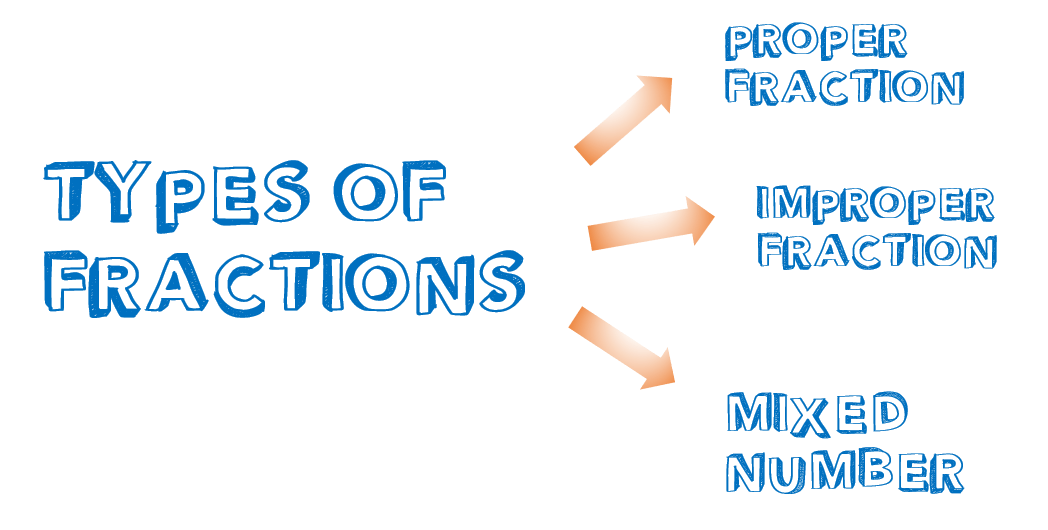These types of fractions can be identified easily by just simply comparing the numerators and the denominators of those fractions. However, please be noted that a fraction can be combined with a whole number as well. We will be considering all of these as we proceed with our lesson.

Meanwhile, we can compare also one fraction from another fraction/s. Depending on the value of their denominators, they can be either:

1. Like Fractions (or Similar Fractions)

2. Unlike Fractions (or Dissimilar Fractions)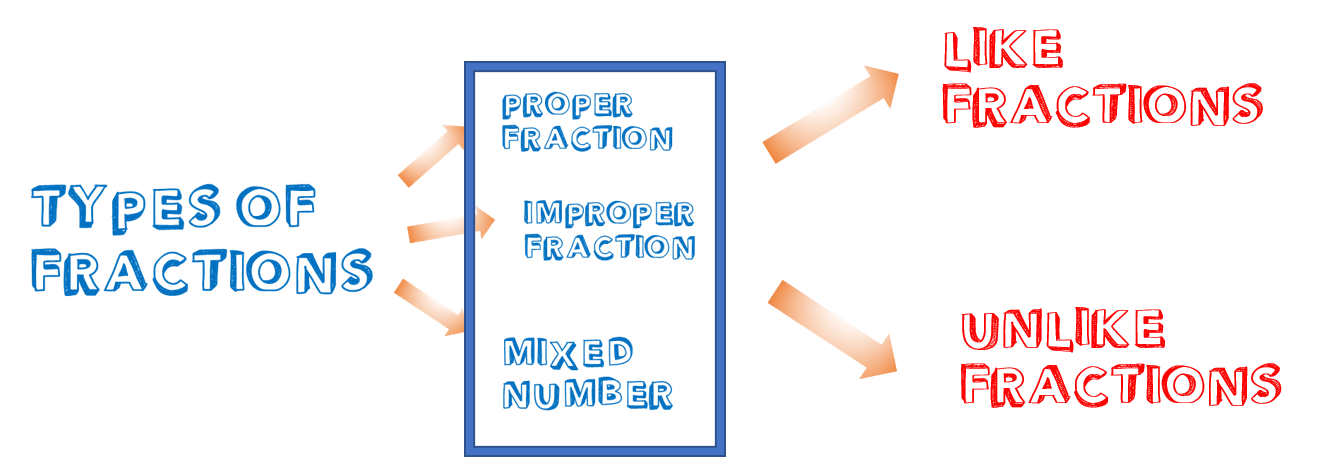Identifying a group of fractions is very important especially when doing addition and subtraction of fraction.

This lesson will prepare you for further fraction-related topics.

Let’s get started.

### What is a Proper Fraction?

When the numerator is less than the denominator, the fraction is called proper fraction.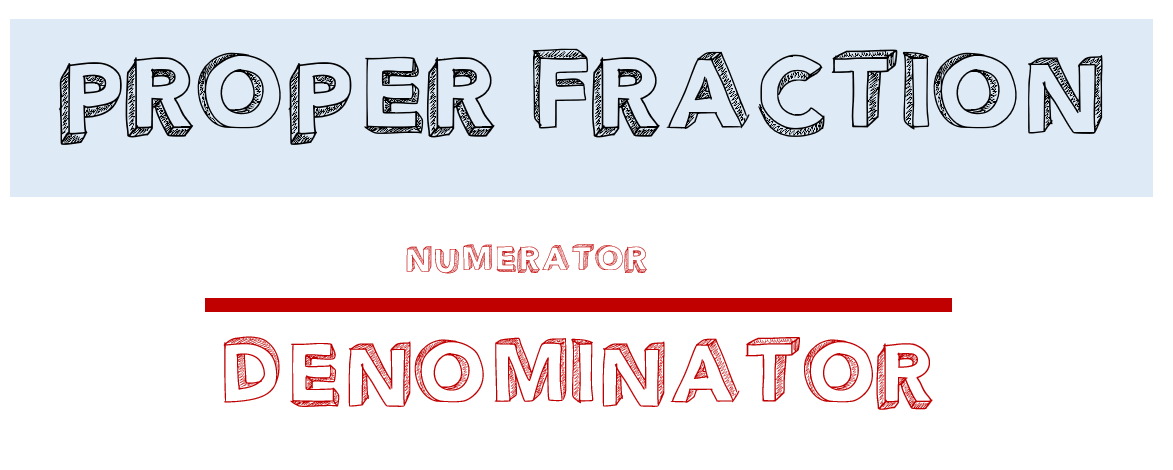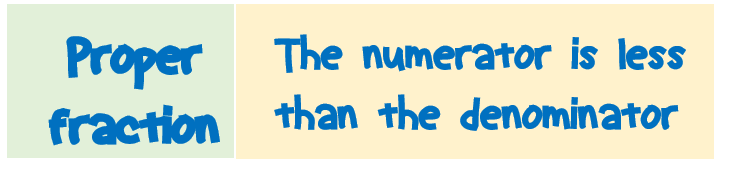1/2, 2/3, 4/7, 3/5 and 3/9 are some examples of proper fractions.

1/2 is a proper fraction because the numerator 1 is less than its denominator 2.

2/3 is also a proper fraction because the numerator 2 is less than its denominator 3.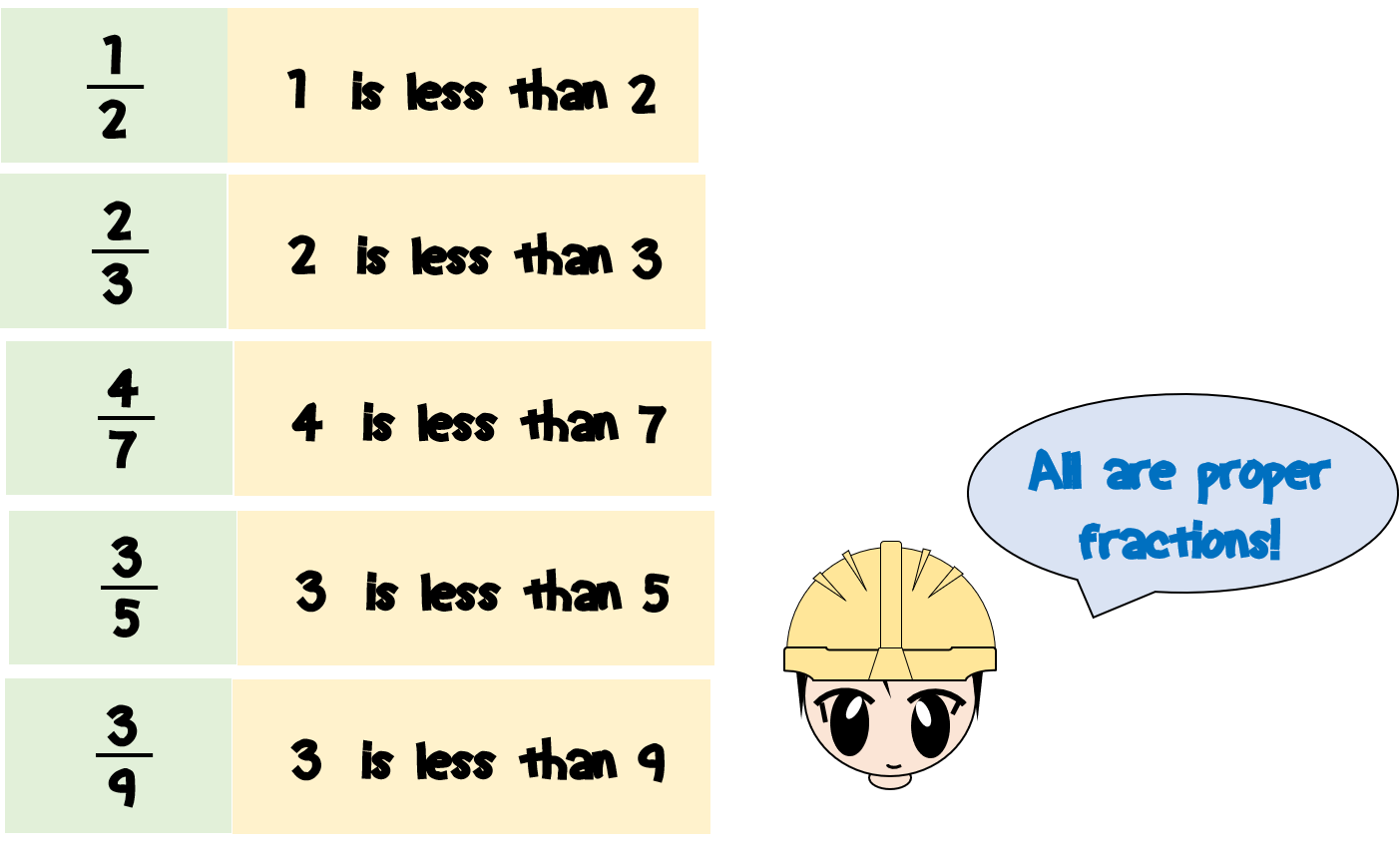All we need to do is to compare the numerator to the denominator of a fraction.

We can come up with infinite numbers of proper fractions.

Important Notes About Proper Fractions (Primary Level):

Note 1. The value of proper fractions is always less than one. To verify this, we can refer to the discussions I made in my previous post entitled “What is a fraction?”. Fraction is actually a division – numerator divided by denominator. For a proper fraction, since the numerator is less than the denominator, the result will be less than one after performing the division.

Note 2. For a proper fraction, zero is not allowed as numerator because we will be defining proper fraction between the values greater than zero and less than one. If we will consider above Note 1, having zero as numerator will result in a zero value. It is because zero divided by any number is equal to zero.

As of now, we will not be dealing with negative numbers to avoid any confusion.

### What is an Improper Fraction?

When a fraction has the numerator greater than the denominator, the fraction is called improper fraction.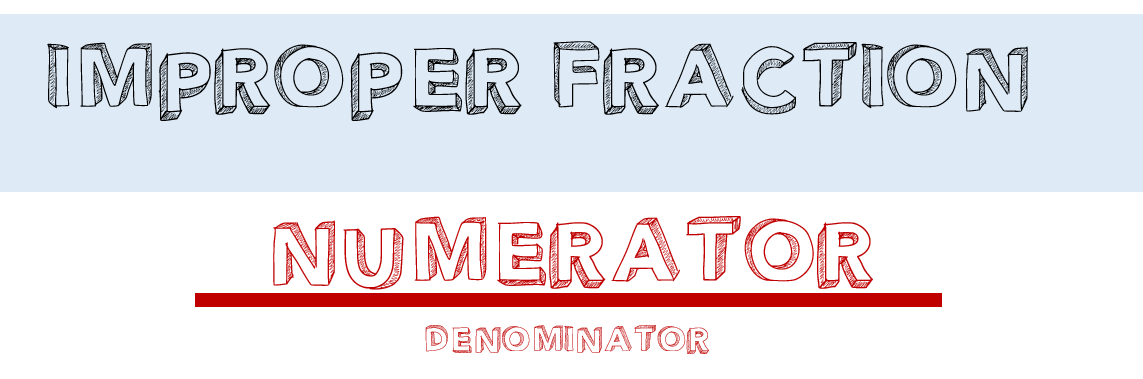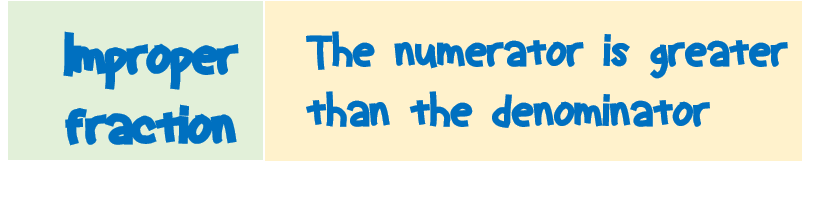5/4, 3/2, 8/3, 5/2, and 10/4 are some examples of improper fractions.

5/4 is an improper fraction because the numerator 5 is greater than its denominator 4.

Also, 3/2 is an improper fraction because the numerator 3 is greater than its denominator 2.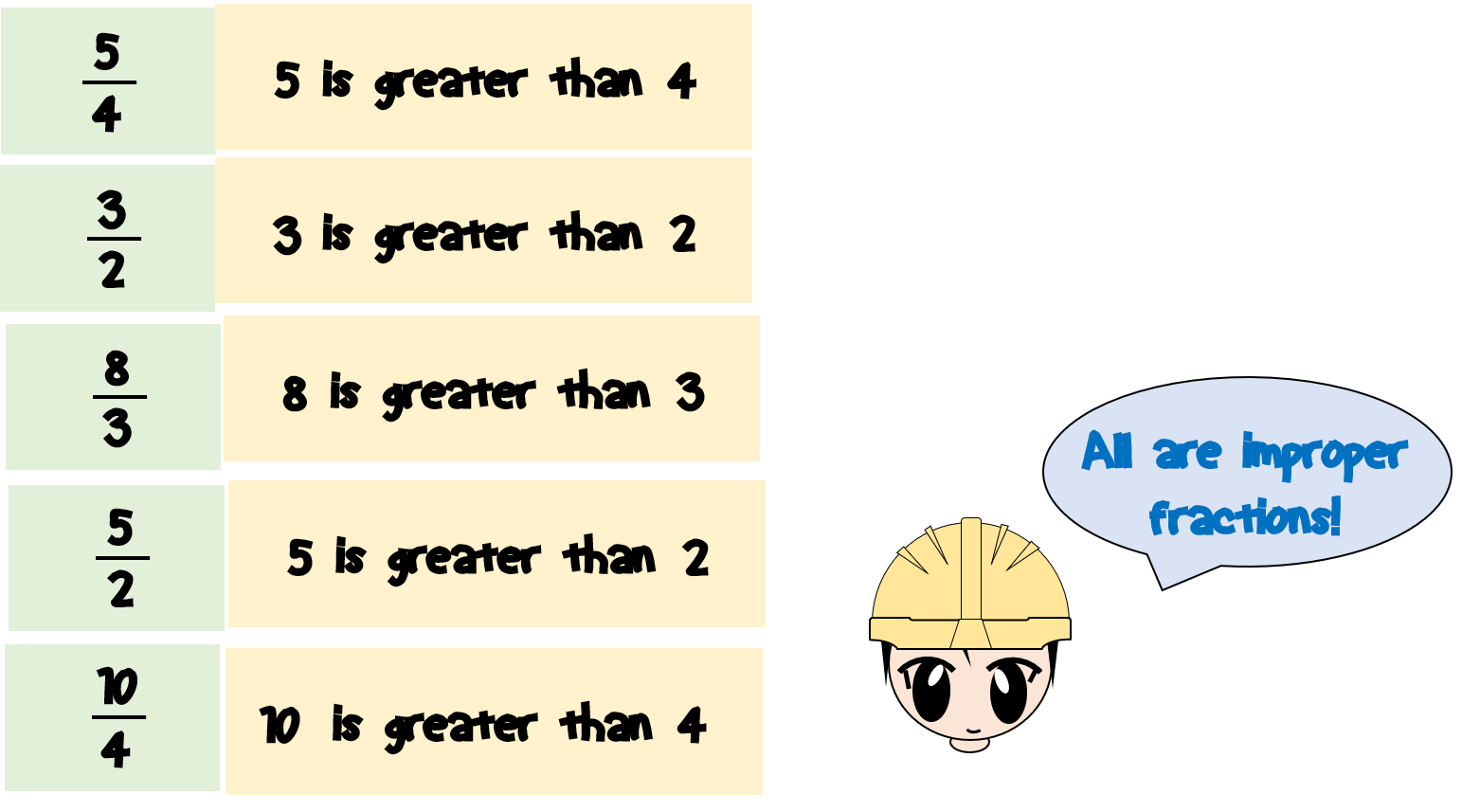Did you see the difference between proper fraction and improper fraction?

Important Notes About Improper Fractions (Primary Level):

Note 1. The value of improper fractions is always greater than one. If we will do the division, since the numerator is greater than the denominator, the result will be greater than one.

Note 2. An improper fraction can be converted into a mixed number. I will be discussing how to convert an improper fraction into a mixed number in my following post.

We will be focusing only on positive numbers at this level.

### What is a Mixed Number?

A mixed number is a number consisting of a proper fraction and a whole number.

It is written as the whole number at the left and the proper fraction at the right.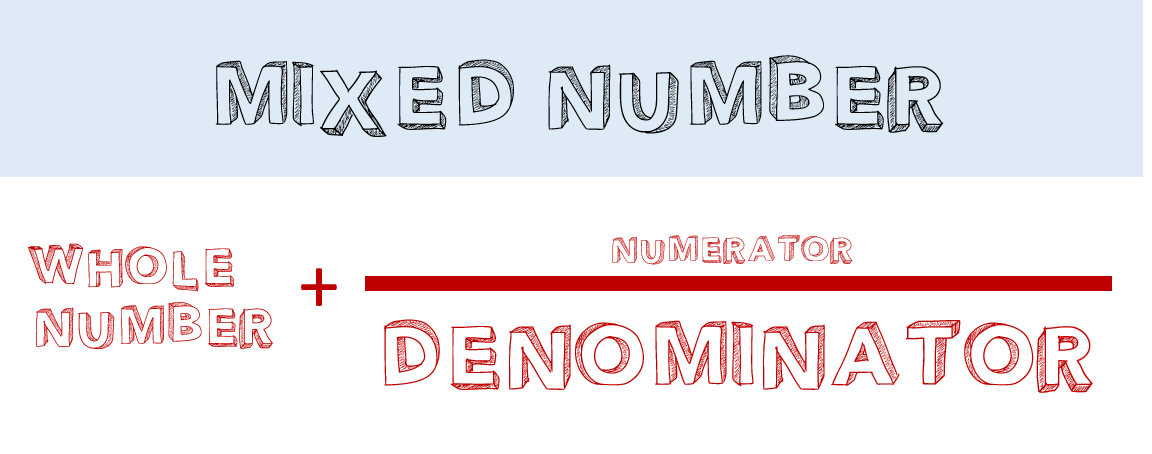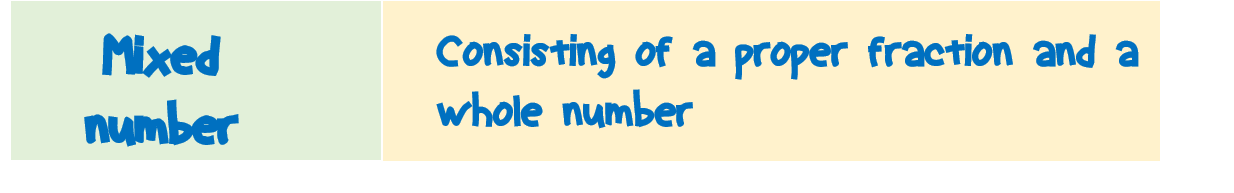1 1/2 is an example of a mixed number. If you can notice, the whole number 1 is at the left side and the proper fraction 1/2 is at the right side. Below are some examples of mixed numbers.

1 3/4 is another example of a mixed number.The value of a mixed number is similar to adding the whole number and the proper fraction. When you add them, it will result in an improper fraction.

Important Notes About Mixed Number (Primary Level):

Note 1. The value of mixed numbers is always greater than one.

Note 2. A mixed number can be converted into an improper fraction. I will be posting a topic related to how to convert mixed numbers into an improper fraction.

### Like and Unlike Fractions

Like Fractions (Similar Fractions)

When two or more fractions have the same denominators, they are called “like fractions”. Like fractions are also called similar fractions.1/4 and 3/4 are like fractions because they have the same denominators. Their denominators are both 4.

1/6, 5/6, and 9/6 are also like fractions. Do you know the reason why?Unlike Fractions (Dissimilar Fractions)

When two or more fractions have different denominators, they are called “unlike fractions”. Unlike fractions are also called dissimilar fractions.1/2 and 1/4 are unlike fractions because they have different denominators. The denominators are 2 and 4 which are not the same.

1/8, 2/3, and 2/5 are also unlike fractions.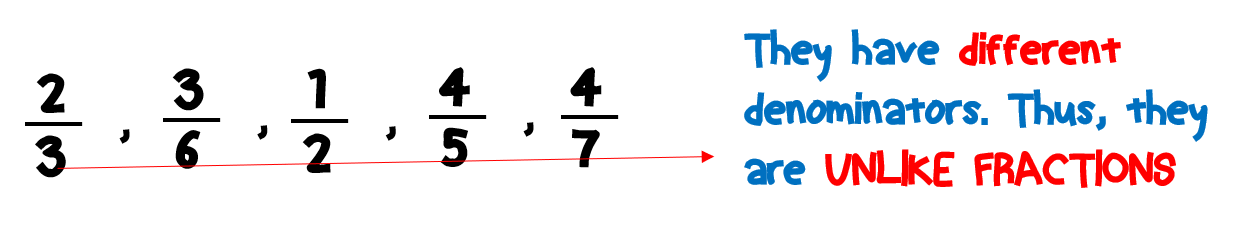Let’s have another example.

What about 2/3, 2/4, and 4/3? Are they like or unlike fractions?

Since the three fractions do not have all the same denominators, we cannot call all of them like fractions (similar fractions). Instead, we can answer like this way:

2/3 and 2/4 are unlike fractions.

2/3 and 4/3 are like fractions.

2/4 and 4/3 are unlike fractions.

Easy. Isn’t it?

### Summary

1. A fraction can be either proper fraction, improper fraction, or mixed numbers.
2. A fraction is a proper fraction if its numerator is less than the denominator, an improper fraction if the numerator is greater than the denominator, and a mixed number if it consists of a proper fraction and a mixed number.
3. Two or more fractions can be either like fractions (similar fraction) or unlike fractions (dissimilar fractions).
4. Like fraction or similar fractions are fractions having the same denominators while unlike fractions or dissimilar fractions are fractions having different denominators.

If you have some questions or clarifications related to the above topic, please feel free to drop those in the comments section below and I will be happy to help you. Enjoy!

### Related Topics

1. What is a Fraction?

2. What are Equivalent Fractions?

1.Demfy says:
2.Salinahasni says: